# pch in R: The different point shapes available in R

The pch in R is “short for plot characters, symbols, or shapes we can use to make plots”. It is an argument used in various plotting functions, such as plot(), points(), and lines(), to specify the type of symbol or marker to be used in the plot.

In R, there are 26 built-in shapes available for use, and they can be identified by numbers ranging from 0 to 25(“+”, “.”, “;”, etc.).

The first 19 (0:18) numbers represent S-compatible vector symbols, and the remaining 7 (19:25) represent the R-specific vector symbols.

The different point symbols commonly used in R are shown below:## pch symbols

1. pch = 0, square
2. pch = 1, circle
3. pch = 2, triangle point up
4. pch = 3, plus
5. pch = 4, cross
6. pch = 5, diamond
7. pch = 6, triangle point down
8. pch = 7, square cross
9. pch = 8, star
10. pch = 9, diamond plus
11. pch = 10, circle plus
12. pch = 11, triangles up and down
13. pch = 12, square plus
14. pch = 13, circle cross
15. pch = 14, square and triangle down
16. pch = 15, filled square
17. pch = 16, filled circle
18. pch = 17, filled triangle point-up
19. pch = 18, filled diamond
20. pch = 19, solid circle
21. pch = 20, bullet (smaller circle)
22. pch = 21, filled circle blue
23. pch = 22, filled square blue
24. pch = 23, filled diamond blue
25. pch = 24, filled triangle point-up blue
26. pch = 25, filled triangle point down blue

You can see the pch numbers from the above section, and R will put the symbol according to that number.

## pch=16

The point symbol pch = 16 is a filled circle. Therefore, the pch = 16 generates the filled circle figure in the plot.

## pch 19

The point symbol pch = 19 is a solid circle. Therefore, pch 19 generates the solid circle figure in the plot.

## Example 1: Use the pch = 10

Let’s use the equation y = x^3. It means we will define two vectors, x and y. The y is the cube of x.

``````x <- c(1, 2, 3, 4, 5, 6, 7)
y <- c(1, 8, 27, 64, 125, 216, 343)``````

Let’s plot the y = x^3 values.

``````x <- c(1, 2, 3, 4, 5, 6, 7)
y <- c(1, 8, 27, 64, 125, 216, 343)

plot(x, y)``````

Output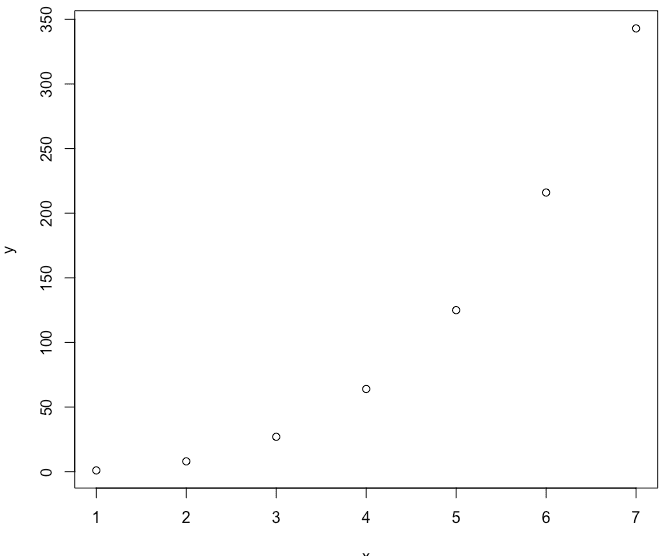The default character for the plot is a circle.

To change that circle symbol, you can pass the pch with a proper argument ranging from 0 to 26.

Let’s pass pch = 10.

``````x <- c(1, 2, 3, 4, 5, 6, 7)
y <- c(1, 8, 27, 64, 125, 216, 343)

plot(x, y, pch=10)``````

Output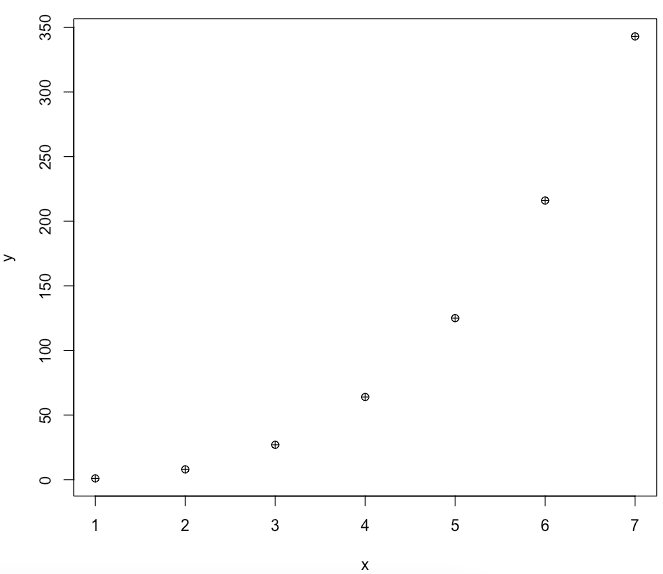## Example 2: Change background color and size of pch

One of the most prominent features of the pch codes is that you can modify their background fill color and their borderline type, color, size of the symbol, and line width of the plotting symbols.

1. col: It is used for color (you can use the color code or color name) for the points.
2. bg: It is used in the background (or fill) color for the open plot symbols. It can be used only when pch ranges from 21 to 25, including 21 and 25.
3. cex: It is the size of pch symbols.
4. lwd: It is the line width for the plotting symbols.

Let’s pass all these parameters to the plot() function and see the output.

``````x <- c(1, 2, 3, 4, 5, 6, 7)
y <- c(1, 8, 27, 64, 125, 216, 343)

plot(x, y, pch=22, col = "darkgreen", bg = "yellow", cex = 1.9, lwd=2)``````

Output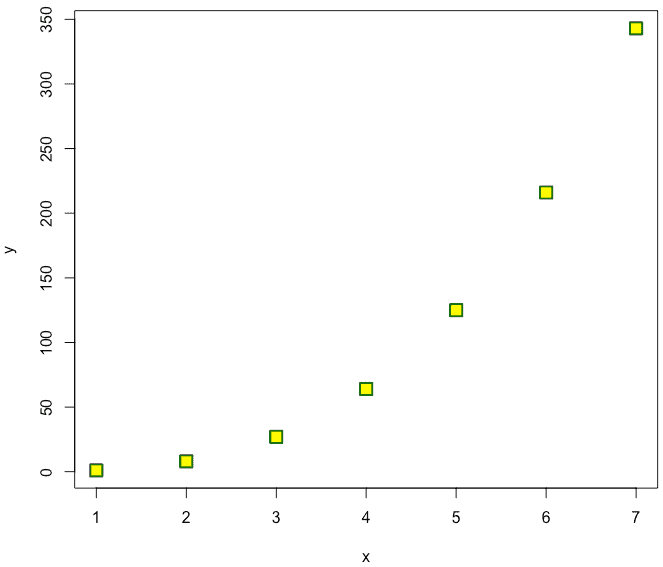## Example 3: Using a built-in dataset

R has many built-in datasets; we will use the iris dataset in this tutorial.

To get the specific part of the object, use the head() function.

``head(iris)``

Output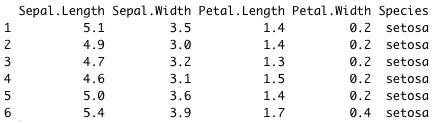We will create two plots and add properties like cex, xlab, ylab, lwd, pch, and col.

``````par(mfrow = c(1, 2))

plot(x = iris\$Sepal.Length, y = iris\$Sepal.Width,
xlab = "Sepal Length", ylab = "Sepal Width",
pch = 24, col = "darkgreen", bg = "yellow",
cex = 1, lwd = 1.3, frame = FALSE)

# Change plot symbol to pch = 15 (filled square)
plot(x = iris\$Sepal.Length, y = iris\$Sepal.Width,
xlab = "Sepal Length", ylab = "Sepal Width",
pch = 15, col = "red",
cex = 1.5, lwd=1.3, frame = FALSE)

``````

Output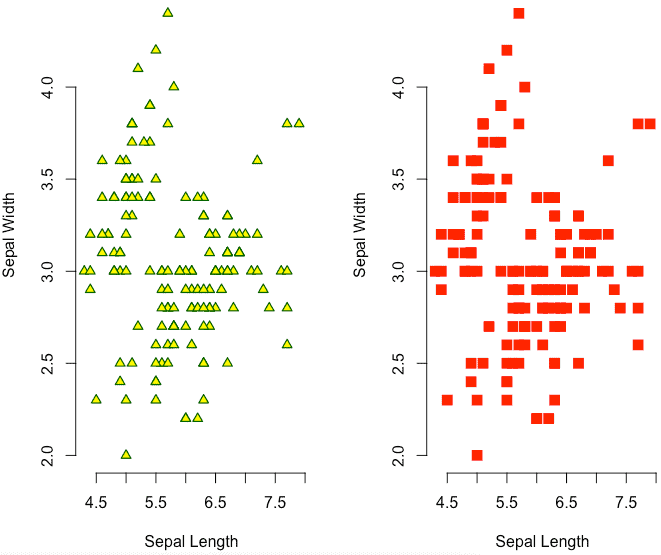## Example 4: Applying a legend to a plot

``````# Colors
colors <- c("red", "darkgreen", "blue")
colors <- colors[as.numeric(iris\$Species)]

# Shapes
shapes = c(22, 23, 24)
shapes <- shapes[as.numeric(iris\$Species)]

# Plot
plot(x = iris\$Sepal.Length, y = iris\$Sepal.Width, frame = FALSE,
xlab = "Sepal Length", ylab = "Sepal Width",
col = colors, pch = shapes)

# Legend
legend("topright", legend = levels(iris\$Species),
col = c("red", "darkgreen", "blue"),
pch = c(22, 23, 24) )

``````

Output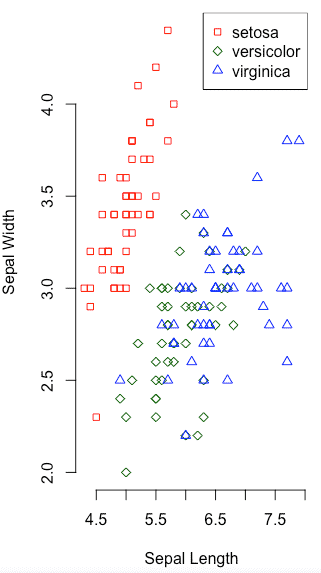That’s all!